Home MonkeyNotes Printable Notes Digital Library Study Guides Study Smart Parents Tips College Planning Test Prep Fun Zone Help / FAQ How to Cite New Title Request

Here y = x2 - 2

\ y + D y = ( x + D x )2 - 2

= x2 + 2 x D x + (D x)2 - 2

\ D y = ( x2 + 2 x D x + (D x)2 - 2) - (x2 - 2)

= 2 x D x + (D x)2

and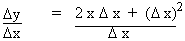= 2 x + (D x)

Interpretation In the figure above, PS is parallel to x - axis and QS is parallel to y - axis. If the secant PQ has inclination µ with positive of x - axis, then tan µ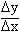which is the slope of secant PQ.

Now,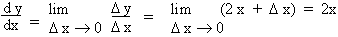Interpretation The limiting position of the secant PQ at P is the tangent to the curve y = x2 - 2 at P. i.e. Q ® P then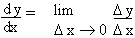which is the slope of tangent at P to y = x2
- 2Your browser does not support the IFRAME tag.

Example 9 If s = 3 t2 + 7 is the distance moved by a body along a straight line from a fixed point o in time t (1) find Ds in s when t varies from t = t0 to t = t0 + D t (2) find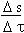and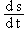and interpret the results.

(1) Here s = 3 t2 + 7,

then s + D s = 3(t + D t)2 + 7

= 3 t2 + 6 t D t + 3(D t)2 + 7

and
Ds = 6 t D t + 3 (D t)2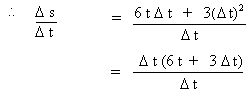= 6 t + 3 D t

Since Ds is the distance moved by a body in Dt ,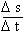is the average rate of change of distance with respect to time. In other words it is the average velocity of the body in t to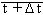Index

4. 1 Derivability At A Point
4. 2 Derivability In An Interval
4. 3 Derivability And Continuity Of A Function At A Point
4. 4 Some Counter Examples
4. 5 Interpretation Of Derivatives
4. 6 Theorems On Derivatives (differentiation Rules)
4. 7 Derivatives Of Standard Functions
4. 8 Derivative Of A Composite Function
4. 9 Differentiation Of Implicit Functions
4.10 Derivative Of An Inverse Function
4.11 Derivatives Of Inverse Trigonometric Functions
4.12 Derivatives Of Exponential & Logarithmic Functions
4.13 Logarithmic Differentiation
4.14 Derivatives Of Functions In Parametric Form
4.15 Higher order Derivatives

Chapter 5